# Capital Budgeting – Financial and Strategic Management MCQ

0

Capital Budgeting – Financial and Strategic Management MCQ

1 / 53

A project has an expected NPV of 1,22,000, Its coefficient of variation is 0.73 77. The risk-free rate is 8% and the cost of capital is 10%. What is the standard deviation of the project?

2 / 53

Z Ltd. is considering a project with the following cash flow:Cost of the plant: 70,000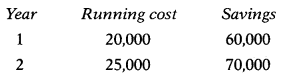The cost of capital is 8%. Measure the sensitivity of the project to the change in the level of savings.
PVAF(8%, 1): 0.926
PVAF (8%, 2): 0.857

3 / 53

X Ltd. is considering a project with the following cash flow:Cost of the plant: 70,000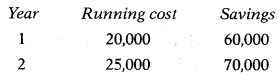The cost of capital is 8%. Measure the sensitivity of the project to the change in the level of plant cost.
PVAF(8%, 1): 0.926
PVAF (8%, 2): 0.857

4 / 53

Following forecasts are made about a proposal which is being evaluated by a firm:Initial outlay: ₹ 1,50,000
Life: 4 years
CFAT: ₹ 56,250
PVAF(14%, 3): 2.3216
PVAF(14%, 4): 2.9137
Calculate the sensitivity of the annual cash flows.

5 / 53

A project is expected to generate a CFAT of ₹ 3,56,000. Other data relating to the project are as follows:Useful life = 6 years.
Risk free rate = 8%
Certainty factor = 0.9 Tax rate = 35%
Initial investment = ₹ 12,00,000.
Depreciation Method = SLM.
Calculate risk-adjusted IRR of the project using rates of 8% & 16%.

6 / 53

The following data is available for Project P:

 NPV (₹ in Crore) Probability 15 0.1 30 0.4 60 0.4 75 0.1Calculate co-efficient of variation for Project P.

7 / 53

Laxmi Ltd. is considering a proposal to buy one of the two machines. Each machine requires an investment of 134,375 and has a useful life of 12 years. Estimates associated with these two machines are as follows: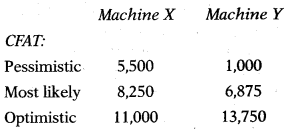The company’s cost of capital is 15% and the risk-free borrowing rate is 6%. Which of the following statement is correct?

8 / 53

X Ltd. is considering to start a new project for which it has gathered the following data:

 NPV (₹ in lakh) Probability 32 0.4 44 0.4 57 0.2Compute the risk associated with the project.

9 / 53

A company has 15,00,000 available for investment and is considering the following four divisible, but not repeatable, projects to invest in: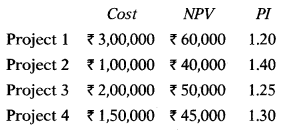What is the maximum net present value the company can generate from its investment?

10 / 53

Taxmann is considering taking a new project. Management uses the Certainty Equivalent (CE) approach to evaluate the projects. The project is expected to generate a cash flow of ₹ 57,500 for the next 5 years. CE Factors are 0.90,0.85,0.75,0.70 & 0.65. Projects require an initial investment of ₹ 1,50,000. The company’s cost of capital is 12% and the risk-free borrowing rate is 7%. What is the NPV of this project?

11 / 53

A company buys a machine for ₹ 10,000 and sells it for ₹ 2,000 at the end of year 3. Running costs of the machine are:Year 1 = ₹ 3,000
Year 2 = ₹ 5,000
Year 3 = ₹ 7,000
If a series of machines are brought, run, and sold on an infinite cycle of replacements, what is the equivalent annual cost of the machine if the discount rate is 10%?

12 / 53

Following data are furnished by XY Leasing Ltd.:

 Investment cost ₹ 1,125 lakhs Primary lease term 5 Years Residual value NIL The pre-tax required rate of return 25%The company wants to charge stepped lease rentals (an annual increase of 15%).Lease rental for the 3rd year =?

13 / 53

Agrani Ltd. is in the business of manufacturing bearings. Some more product lines are being planned to be added to the existing system. The cost of the machine is ₹ 40,00,000 having a useful life of 5 years with a salvage value of ₹ 8,00,000. The full purchase value of the machine can be financed by a 20% loan repayable in 5 equal installments falling due at the end of each year. Calculate the figure of interest payable at the end of the 5th year.

14 / 53

A company can obtain an asset on lease by paying 5 equal lease rentals annually. Such lease rentals are payable at the beginning of the year. The leasing company desires a return of 10% on the gross value of the asset. The tax rate is 40%. The cost of capital is 9%. The cost of the asset is ₹ 7,61,790. Calculate the lease rental payable by the company each year.

15 / 53

X Ltd. faced with the decision to purchase or acquire on lease a machine. The cost of the machine is ₹ 5,07,860. The asset can be financed by taking a loan on which interest is payable @15% and the loan will be paid in 5 equal installments inclusive of interest. The tax rate is 40%. Assume loan installment is payable at the beginning of the year. What will be the loan installment amount for each year?

16 / 53

A company is considering three methods of attracting customers to expand its business by undertaking –(A) advertising campaign; (B) display of neon signs; and (C) direct delivery service. The initial Outlay for each alternative is as under:
A ₹ 1,00,000
B ₹1,50,000
C ₹ 1,50,000
If A is carried out, but not B, it has an NPV of ₹ 1,25,000. If B is done, but not A, B has an NPV of ₹ 45,000. However, if both are done, then NPV is ₹ 2,00,000. The NPV of the delivery system C is ₹ 90,000. Its NPV is not dependent on whether A or B is adopted and the NPV of A or B does not depend on whether C is adopted. Which of the investments should be made by the company if the budgeted amount is only ₹ 2,50,000?

17 / 53

Bhaskar Ltd. estimated that a proposed project’s 8-year net cash benefit will be ₹ 4,000 per year for years 1 to 8, with an additional terminal benefit of ₹ 8,000 at the end of the eighth year. Assuming that these cash inflows satisfy exactly the required rate of return of 8 percent, the project’s initial cash outflow is closest to which of the following four possible answers?

18 / 53

The profitability index of Project X is 1.20167 when its cash flow is discounted at 12%. The initial investment in the project was ₹ 1,50,000. This project generates equal cash flow over five years time. How much cash flow will be generated by the project each year?

19 / 53

The following data is available for Project A whose initial investment is ₹ 50,000 and salvage value after 5 years is ₹ 3,750.

 Year CFAT (₹) 1 25,000 2 20,000 3 10,000 4 10,000 5 1,250What is the NPV of Project A if the Ke of the company is 10%? Ignore taxation.

20 / 53

Rakesh Ltd. is considering investing in one of four projects for which an analyst has calculated payback period reciprocal’ as 25%, 40%, 50% & 75% respectively for Project P, Q, R & S. Which project will be selected on ‘payback period’ method of capital budgeting?

21 / 53

A project requires initial investment of ₹ 2,00,000 and estimated to generate cash flow after tax of ₹ 1,00,000, ₹ 80,000, ₹ 40,000, ₹ 20,000 & ₹ 10,000 in next 5 years. What is the payback period of the project?

22 / 53

Which of the following is the correct formula to calculate the coefficient of variation?

23 / 53

Consider the following two statements:1. Risk analysis gives management better information about the possible outcomes that may occur so that management can use their judgment and experience to accept investment or reject it.
2. In relation to capital budgeting, sensitivity analysis deals with the consideration of the sensitivity of the NPV to different variables contributing to the NPV.

24 / 53

Which of the following relate to finance leases as opposed to operating leases?1. At the inception of the lease the present value of the minimum lease payments amounts to at least substantially all of the fan- the value of the leased asset.
2. Ownership of the asset remains with the lessor for the entire lease period.
3. Asset acquired under a finance lease is shown as an asset in the balance sheet of the lessee.

25 / 53

What technique is best used when cash flows are related to cash flows in previous periods?

26 / 53

The probability associated with the first portion of a complete branch of the probability tree is referred to as the

27 / 53

A firm that ignores risk differences (does not adjust for risk) when choosing new investment projects will generally –

28 / 53

A ____approach to examine project risk occurs when cash flows are arranged such that a cash flow in one period leads to several possible cash flow outcomes in the subsequent period. Each individual cash flow in the subsequent period then leads to several possible cash flow outcomes in its subsequent period. This process continues numerous times to generate a complete risk-return graphic.

29 / 53

A risk-averse investor is considering four mutually exclusive investments, which have the following characteristics: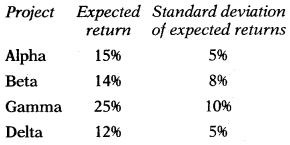Which two of the above investments will the investor immediately reject?

30 / 53

The coefficient of variation of net present value measures the

31 / 53

Which of the following is the correct formula to calculate the risk-adjusted discount rate?

32 / 53

Two mutually exclusive projects are being considered. Neither project will be repeated again in the future after their current lives are complete. There exists a potential problem through – the expected life of the first project is one year and the expected life of the second project is three years. This has caused the NPV and IRR methods to suggest different project preferences. What technique can be used to help make a better decision in this scenario?

33 / 53

Which one of the following projects – A, B, C, or D – should be accepted? The expected return on the market is 16% and the risk-free rate is 6%.

34 / 53

Assume that a firm has accurately calculated the net cash flows relating to two mutually exclusive investment proposals. If the net present value of both proposals exceed zero and the firm is not under the constraint of capital rationing, then the firm should

35 / 53

Consider the following statements:(1) Expected value of cash flows is equal to the arithmetic average of the cash flows.
(2) In the case of capital budgeting, the higher the standard deviation better the project is.
(3) In the case of dependent cash flows, the risk is measured with reference to joint probabilities.

36 / 53

Money Discount Rate is equal to:

37 / 53

If two projects are completely independent (or unrelated), the measure of the correlation between them is:

38 / 53

You are considering two mutually exclusive investment proposals, project A and project B. B’s expected value of net present value is \$1,000 less than that for A and A has less dispersion. On the basis of risk and return, you would say that:

39 / 53

The investment proposal with the greatest relative risk would have:

40 / 53

The decision-tree approach is used in:

41 / 53

Which of the following statements is correct regarding the internal rate of return (IRR) method?

42 / 53

When operating under a single-period capital-rationing constraint, you may first want to try selecting projects by descending order of their____in order to give yourself the best chance to select the mix of projects that adds most to the firm value.

43 / 53

A project whose acceptance does not prevent or require the acceptance of one or more alternative projects is referred to as

44 / 53

What is the difference between economic profit and accounting profit?

45 / 53

Ranking projects according to their ability to repay quickly may be useful to firms:

46 / 53

The shorter the payback period –

47 / 53

Statement I:In the case of capital rationing, a company is compelled to invest in projects having the shortest payback period.
Statement II:The shorter the payback period, the less risky is the project. Therefore, it can be considered as an indicator of risk.

48 / 53

When choosing among mutually exclusive projects, the project with –

49 / 53

Incorporating flotation costs into the analysis of a project will:

50 / 53

___ is the discount rate that should be used in capital budgeting.

51 / 53

___ is a project whose cash flows are not affected by the accept/reject decision for other projects.

52 / 53

The decision to accept or reject a capital budgeting project depends on –

53 / 53

Capital budgeting is the process___

This site uses Akismet to reduce spam. Learn how your comment data is processed.